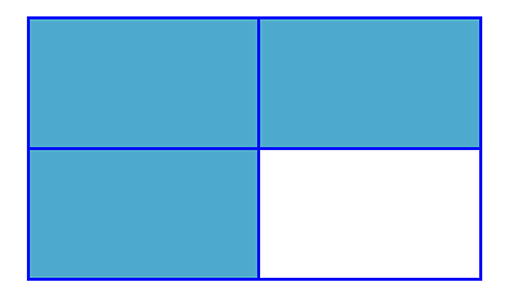Teaching mathematics

Start this free course now. Just create an account and sign in. Enrol and complete the course for a free statement of participation or digital badge if available.

Free course

# 2.1 What are fractions?

Fractions are numbers made of two integers (positive and negative whole numbers), one acting as the numerator and one acting as the denominator.

(Fractions typically refer to quantities which are smaller than 1. In this case the numerator is smaller than the denominator. Fractions with a numerator larger than the denominator have a value greater than 1. They can also be written as mixed numbers; the whole number part and the fraction part).

Consider the fraction illustrated in the diagram below which shows of a rectangle shaded. The rectangle has been divided into 4 equal parts and 3 of these parts have been shaded.Figure 2 A rectangle shaded to show three-quarters

Three- quarters of any quantity can be found using the same process. is found (by dividing by 4 to calculate four equal parts), and then multiplied by 3 to give . This is referred to as a part-whole relationship (Figure 2).

However the number can also be taken to mean that 3 has been divided into 4 equal parts and in this case the value of the fraction is equal to the numerator divided by the denominator. This interpretation of the fraction is used when converting fractions to their equivalent decimals. If you input into a calculator you will get 0.75, which is the decimal equivalent to . This takes the meaning of a quotient (the result of dividing one number by another).

Essentially a fraction such as can have two meanings (the whole-part relation and the quotient) and this can cause difficulty in understanding for some students. One way to address this difficulty is to tell students, for example, that means 3 lots of of something, and it can also mean . This is not the only such case in mathematics, where symbolic notation can take on different meanings and which causes difficulties in students’ understanding.

TM_1